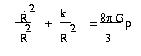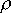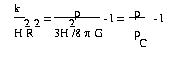QUESTION #252

# How was the critical density of the universe calculated?This equation determines how the scale factor of the universe (essentially the size of the universe), R, changes with time in terms of the energy density,, and k, a measure of the curvature of the universe. The first term in this equation is just the square of the Hubble parameter, H. We now just rearrange this equation: